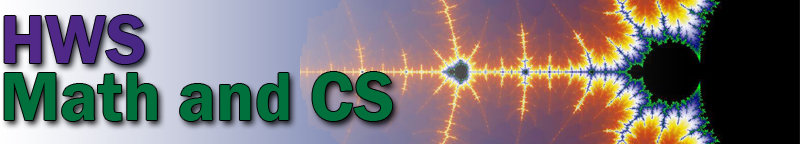Schedule of Courses for Fall 2020 Note: some classes are taught fully remotely but will still have some interaction at scheduled times. Period 1 MWF 8:40–9:40 CPSC 441: Computer Networking, Chris Fietkiewicz Math 130: Calculus I, Alden Gassert       (Lab Tuesday, 8:30–10:00) Period 2 MWF 9:50–10:50 CPSC 343: Database Theory and Practice, Stina Bridgeman Math 100: Elementary Functions, Yan Hao       (Lab Thursday,10:10–11:40) Math 130: Calculus I, Alden Gassert       (Lab Tuesday, 10:10–11:40) Math 204: Linear Algebra, David Eck Period 3 MWF 11:00–12:00 CPSC 329: Software Development, Stina Bridgeman       (Lab Tuesday, 10:10–11:40) Math 130: Calculus I, William Simmons       (Lab Tuesday, 11:50–1:20) Math 131: Calculus II, Yan Hao       (Lab Thursday, 11:50–1:20) Period 4 MWF 12:10–1:10 CPSC 124: Introductory Programming, Hanqing Hu       (Lab Thursday, 10:10–11:40) Math 130: Calculus I, William Simmons       (Lab Tuesday, 1:30–3:00) Period 5 MWF 1:20–2:20 CPSC 124: Introductory Programming, Hanqing Hu       (Lab Thursday, 1:39–3:00) Math 232: Multivariable Calculus, Jennifer Biermann Math 331: Foundations of Analysis, David Eck Period 5A MW 1:20–2:50 Math 114: Mathemathics for Informed Citizenship, Jon Forde Period 7 TuTh 8:30–10:00 Math 278: Number Theory, Joseph Rusinko Period 9 TuTh 11:50–1:20 CPSC 220: Computer Architecture, Chris Fietkiewicz       (Lab Thursday, 1:20–2:50) Period 11 TuTh 3:10–4:40 Math 350: Probability, Jon Forde

 Tentative Schedule of Courses for Spring 2021 Period 1 MWF 8:40–9:40 CPSC 371: Advanced Topics in Computer Science, Chris Fietkiewicz Math 130: Calculus I, Alden Gassert       (Lab Tuesday, 10:10–11:40) Period 2 MWF 9:50–10:50 CPSC 124: Introductory Programming, David Eck       (Lab Tuesday, 11:50–1:20) Math 130: Calculus I, Jocelyn Bell       (Lab Tuesday, 11:50–1:20) Math 131: Calculus II, Jennifer Biermann       (Lab Thursday, 11:50–1:20) Math 135: First Steps into Advanced Math, William Simmons Period 3 MWF 11:00–12:00 CPSC 225: Intermediate Programming, Hanqing Hu       (Lab Thursday, 1:30–3:00) Math 130: Calculus I, Jocelyn Bell       (Lab Tuesday, 1:30–3:00) Math 131: Calculus II, Jennifer Biermann       (Lab Thursday, 1:30–3:00) Period 4 MWF 12:10–1:10 CPSC 229: Foundations of Computation, David Eck Math 237: Differential Equations, Thomas Gassert Period 5 MWF 1:20–2:20 CPSC 327: Data Structures and Algorithms, Hanqing Hu Math 232: Multivariable Calculus, William Simmons Math 375: Abstract Algebra, Jennifer Biermann Period 6 MWf 3:00–4:30 Math 471: Mathematics Capstone, Jon Forde Period 8 TuTh 10:10–11:40 CPSC 441: Artificial Intelligence, Chris Fietkiewicz Math 204: Linear Algebra, Yan Hao Period 10 TuTh 1:30–3:00 Math 351: Mathematical Statistics, Yan Hao Period 11 TuTh 3:10–4:40 Math 114: Mathemathics for Informed Citizenship, Jon Forde